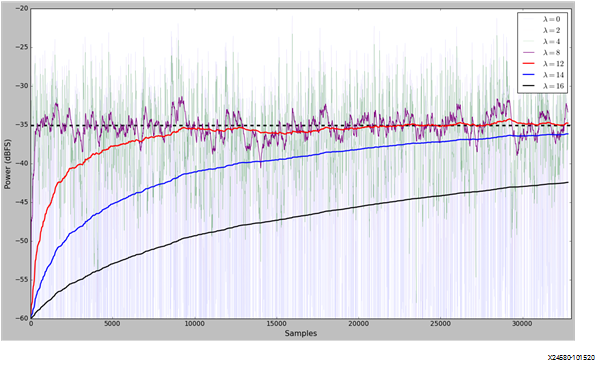# Leaky Integrator - 2.6 English

## Zynq UltraScale+ RFSoC RF Data Converter Gen 1/2/3/DFE LogiCORE IP Product Guide (PG269)

Document ID
PG269
Release Date
2022-10-21
Version
2.6 English

A leaky integrator is used to measure the averaged signal magnitude over a period of time. The leaky integrator time constant is programmable from a single T4/T8 clock cycle to 216 clock cycles.

The transform function of the leaky integrator is showed as following:

• y[n]=(1-1/2λ)y[n-1]+1/2λ |x[n]|

where:

y[n] is the output of leaky integrator

y[n-1] is the last output of leaky integrator

x[n] is the current input value; the output from input stage

2λ is the time constant.

The following table gives the programmable time constant values:

Table 1. Time Constant Table
Lambda Index Lambda Value Time Constant (T4/T8 Cycle)
0 0 2^0
1 2 2^2
2 4 2^4
3 8 2^8
4 12 2^12
5 14 2^14
6 16 2^16
7 N/A N/A
Figure 1. Time ConstantA Gauss distribution signal is used for illustration in above figure. A set of curves base on different time constant shows the 20*log10 outputs of the leaky integrator.

A user programmable flush bit can be used to flush the leaky integrator which reset the value to 0.